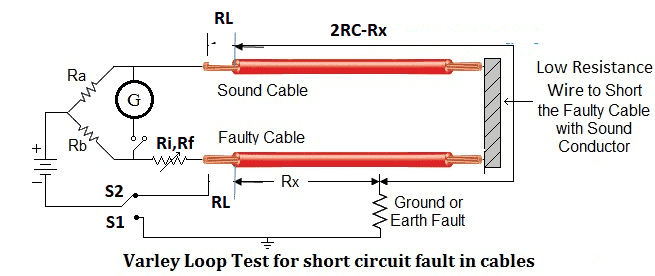# Varley loop R=pL/A formula

## Homework Statement

A Varley Bridge is connected to a faulty three-core copper cable by two identical copper leads of resistance Rl.

(a) Show that for the initial reading (connection to earth);

2Rx = 2Rc – Ri .............................. 1

where Rc is the resistance of the cable core Ri is the initial reading of the bridge
Rx is the cable resistance to the fault from the bridge

2Rc = R f – 2Rl ..............................  2

where Rl is a lead resistance
Rf is the final reading resistance.

Then by substituting (2) in (1) and rearranging the equation, show:

Rx + Rl =  R f R–f Ri  Rc + Rl 

(b) By multiplying the rhs brackets and collecting terms, show the effect of the leads is given by:

R = Rf–Ri R – Rl Ri

x c Rf
Rf
i.e. = effect with no leads – ratio of initial and final readings

(c) Determine the distance to the fault by modifying the expression in (b) and using Rx = x

Rc L

where x is the cable distance to the fault and L is the length of a cable core.

Derive an expression for x, the distance to the fault.

(d) Using R=ρL/A

where ρ is the resistivity,
L is the length
and A is the cross-sectional area of a cable core
show that
Rl=RcAcLl/AlLc

## The Attempt at a Solution

I can work out all the way to section d, but then have no idea what formula to start with

It would help if you provided a diagram with the various resistances labelled. I remember seeing a Varley bridge many years ago, but I don't remember the basic circuitry and how it is connected to cable.This is how the varley bridge circuit looks.
I just cannot see which of my existing formulae or how to create a new to show Rl=RcAcLl/AlLc

#### Attachments

In a) take Ra=Rb. Point b) it is very difficult to guess what the actual formulae are [what is written it is only gibberish].

Point d) I guess Lc=cable length, LL=lead length and ρc=ρL=ρ

Rc=ρ.Lc/Ac ;ρ=Rc.Ac/Lc; RL=ρ.LL/AL